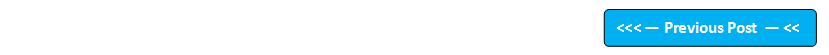## Blog Pages

### 53. Operator PrecedenceThe following row shows the order of precedence for Java operators:

 Highest ( ) ! * / % + - > >= < <= == != & | ?: = Lowest

As its not possible to go through the examples of how each and every operator is having priority over other operator, I'm going to example the operator precedence with  only one example.

 Program to demonstrate the difference before and after using the high priority operator '( )' class OperatorPrecedence {    public static void main(String args[])    {       int a=3,b=4,c=5,d,e;       d=a*b+c;    // without using high priority operator '( )' -> a*b+c i.e. 3*4+c i.e. 12+c i.e. 12+5 i.e. 17       e=a*(b+c);  //After using high priority operator '( )' -> a*(b+c) i.e. a*(4+5) i.e. a*9 i.e. 3*9 i.e. 27       System.out.println("Without using the parenthesis the result is "+d);       System.out.println("After using the parenthesis the result is "+e);    } } Output of this program: Without using the parenthesis the result is 17 After using the parenthesis the result is 27